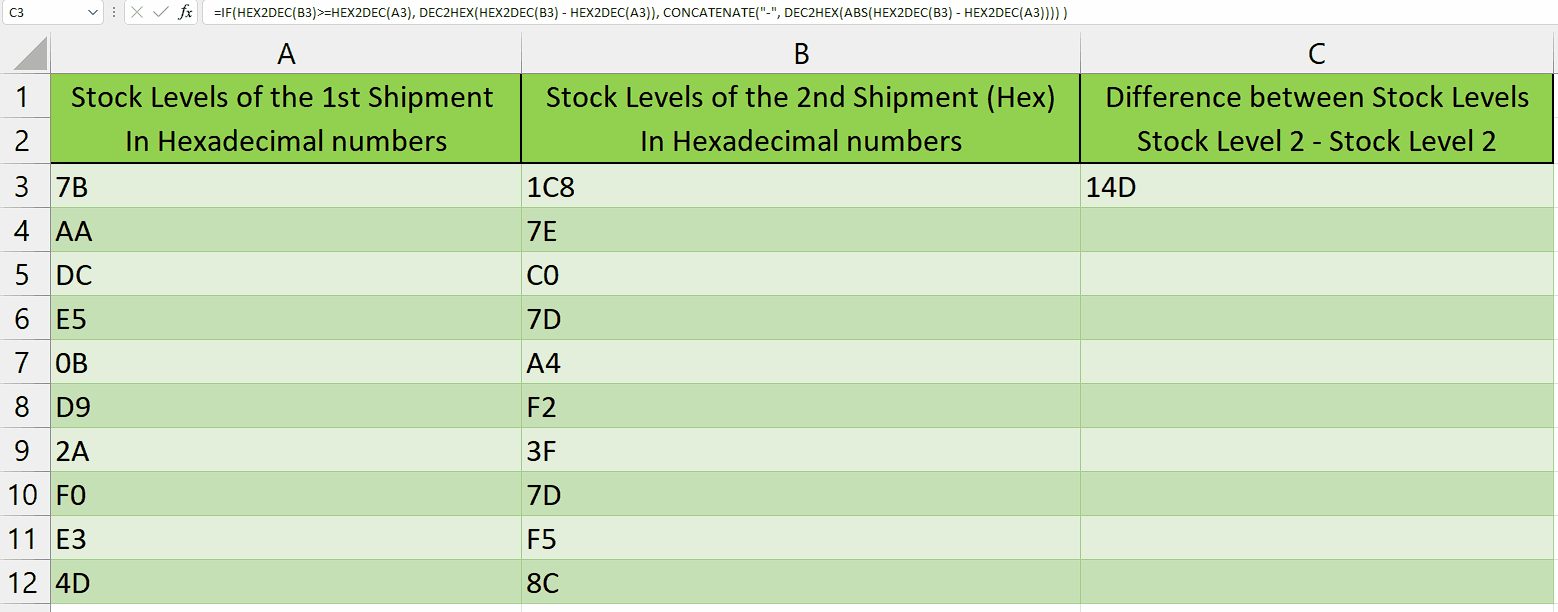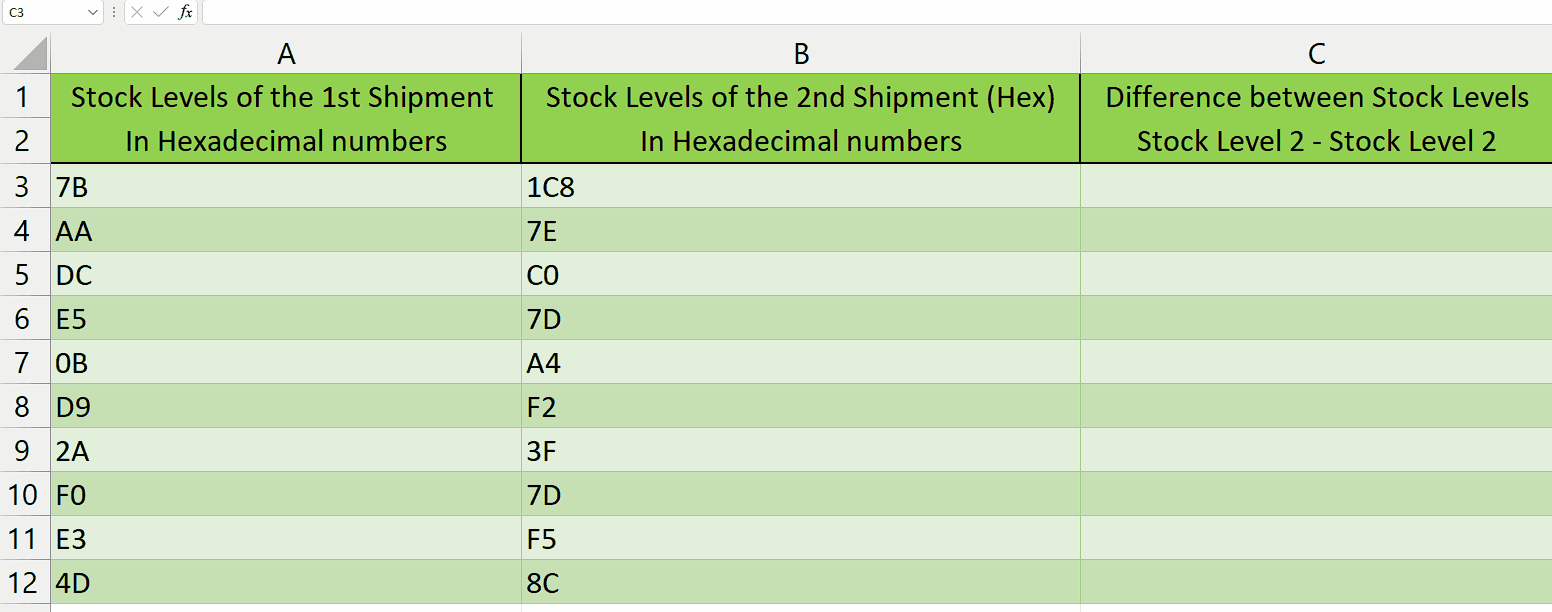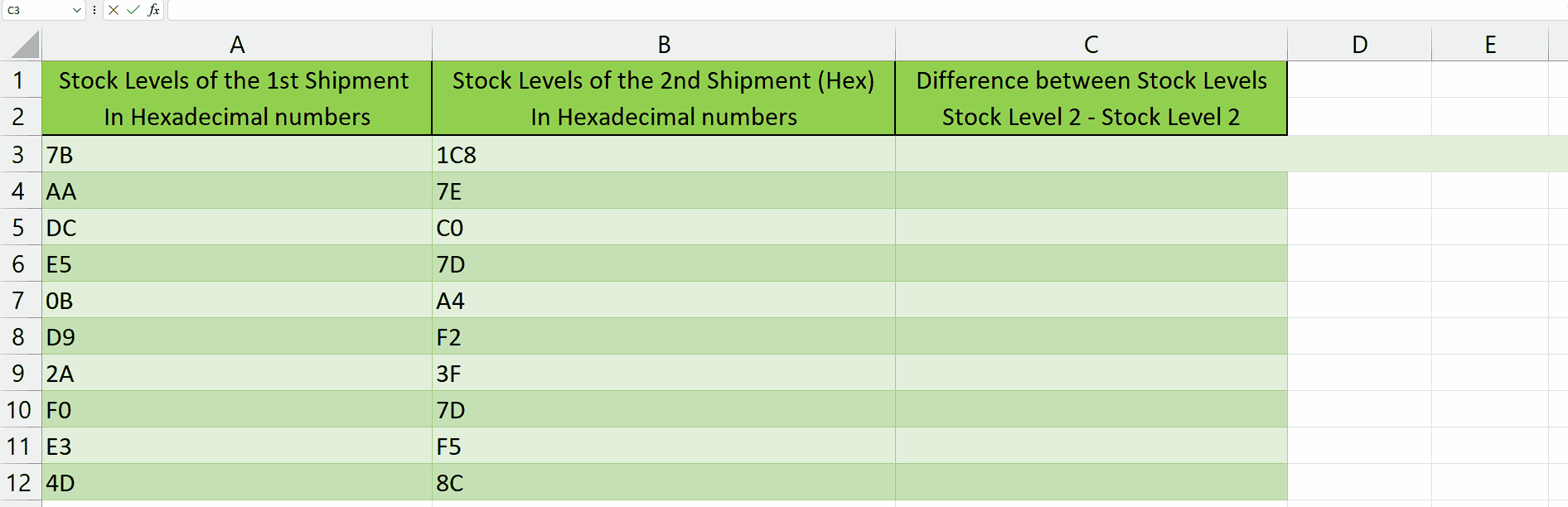# How to subtract Hex numbers in Excel

Imagine you are the inventory manager of a diverse store, responsible for tracking and maintaining stock levels. To monitor the variance between two distinct shipments of products, you decide to utilize hexadecimal numbers. By employing this numerical system, you can effectively manage and measure the differences in stock levels, enhancing inventory control and accuracy. By taking this dataset as an example we will learn how to subtract hex numbers.Hexadecimal subtraction is a crucial and foundational operation extensively utilized in the realm of computer science and digital systems. It plays a pivotal role in enabling a wide range of calculations, data manipulation, and addressing operations. This operation is essential for performing intricate mathematical computations, manipulating binary data, and facilitating precise memory allocation and retrieval.

### Step 1 – Select the cell– Select the cell where you want to display the result of the subtracted hexadecimal numbers.

### Step 2 – Write the formula and implement it– After selecting the cell, we will write this formula in that cell

=IF(HEX2DEC(B3)>=HEX2DEC(A3), DEC2HEX(HEX2DEC(B3) – HEX2DEC(A3)), CONCATENATE(“-“, DEC2HEX(ABS(HEX2DEC(B3) – HEX2DEC(A3)))) )

Let’s break down each component of the formula:

IF(HEX2DEC(B3)>=HEX2DEC(A3), …): This is the conditional statement that checks if the decimal value of the hexadecimal number in cell B3 is greater than or equal to the decimal value of the hexadecimal number in cell A3.

DEC2HEX(HEX2DEC(B3) – HEX2DEC(A3)): If the condition in the first part is true, this part of the formula calculates the difference between the decimal values of B3 and A3 using the HEX2DEC function. It then converts the resulting decimal value back to hexadecimal using the DEC2HEX function.

CONCATENATE(“-“, DEC2HEX(ABS(HEX2DEC(B3) – HEX2DEC(A3)))): If the condition in the first part is false, this part of the formula calculates the absolute difference between the decimal values of B3 and A3 using the HEX2DEC function. It then converts the absolute difference to hexadecimal using DEC2HEX and adds a minus sign in front using the CONCATENATE function.

-After wriing this formula, press Enter and the result will appear in the cell.

### Step 3 – Implementing the formula to the whole range– To apply the formula across a range of cells, begin by selecting the cell containing the desired result, such as cell C3
– Next, navigate to the bottom right corner of the cell until your cursor transforms into a plus (+) shape, known as the fill handle.
– Double-Click on this fill handle and the formula would be applied to the whole range.

## Explanation of the formula used in Step 2:

This formula is an Excel formula that calculates the difference between two hexadecimal numbers and returns the result in hexadecimal format. It uses the functions HEX2DEC, DEC2HEX, and ABS, along with conditional logic.

The formula first converts the values in cell B3 and A3 from hexadecimal to decimal using the HEX2DEC function. It then compares the decimal values using the IF statement. If the value in B3 is greater than or equal to the value in A3, it subtracts the decimal values and converts the result back to hexadecimal using DEC2HEX. If the value in A3 is greater than the value in B3, it subtracts the decimal values, takes the absolute value using ABS, and converts the result to hexadecimal, while adding a minus sign using CONCATENATE.

Using the HEX2DEC and DEC2HEX functions simplifies the process by directly converting hexadecimal values to decimal and vice versa. Manually converting the values would be cumbersome and prone to errors. The formula handles the conversion and subtraction automatically, ensuring accuracy and preventing errors. Additionally, the use of conditional logic allows for different actions to be taken based on the comparison of the values, providing flexibility in the formula’s functionality.